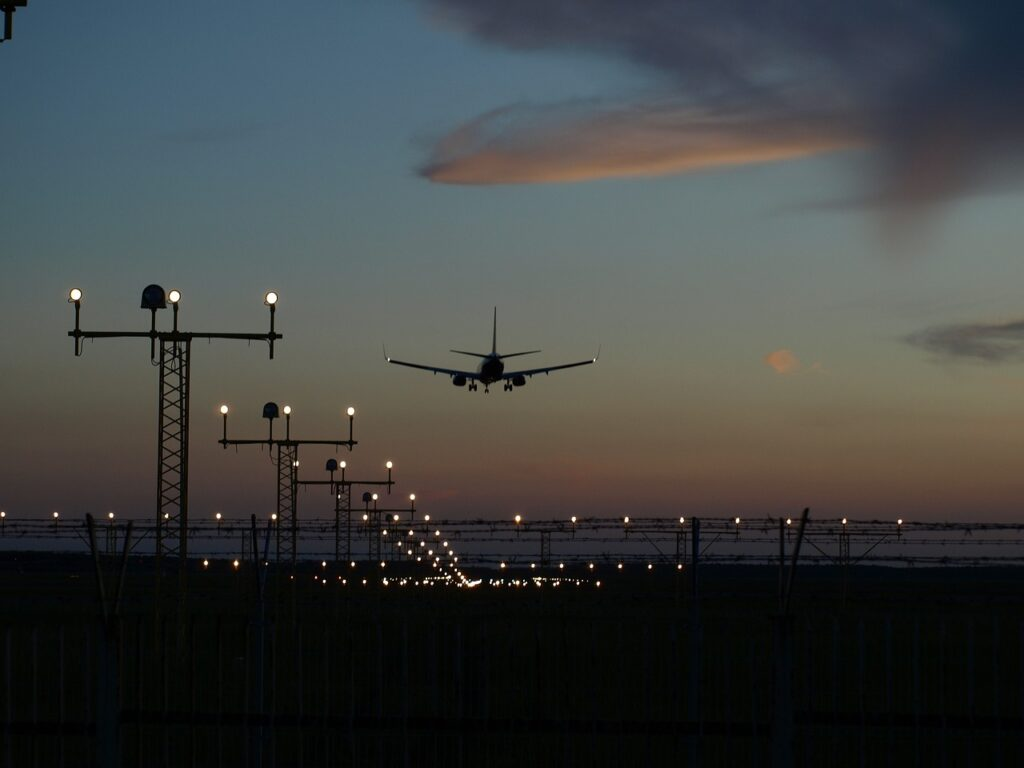#### 365Villas Integrates WhatsApp for Streamlined Vacation Rental Management Communication

September 26, 2023The first quarter of 2023 saw all 26 scheduled U.S. passenger airlines recording their financial results.

# US Airlines Show Progress in First Quarter 2023 with \$1.2 Billion Losses

US Airlines Show Progress in First Quarter 2023 with \$1.2 Billion Losses, Marking an Upturn from First Quarter 2022

The first quarter of 2023 saw all 26 scheduled U.S. passenger airlines recording their financial results. During this period, these airlines reported a net loss of \$1.2 billion after taxes, but managed to achieve a pre-tax operating gain of \$32 million.

Comparatively, in the first quarter of 2022, the airlines had experienced a more significant financial setback. At that time, they reported an after-tax net loss of \$5.1 billion, along with a pre-tax operating loss of \$5.2 billion.

Systemwide:

1. After-tax net income:
• In 1Q 2023, there was a net loss of \$1.2 billion.
• In 4Q 2022, there was a net gain of \$2.1 billion.
• In 1Q 2022, there was a net loss of \$5.1 billion.
2. Pre-tax operating profit:
• In 1Q 2023, there was a pre-tax operating gain of \$32 million.
• In 4Q 2022, there was a pre-tax operating gain of \$3.7 billion.
• In 1Q 2022, there was a pre-tax operating loss of \$5.2 billion.
3. Total operating revenue in 1Q 2023 amounted to \$52.9 billion.
4. Share of total operating revenue in 1Q 2023:
• Fares accounted for \$39.5 billion, representing 74.6% of the revenue (compared to 68.9% in 1Q 2022).
• Baggage fees contributed \$1.7 billion, representing 3.3% of the revenue (compared to 3.7% in 1Q 2022).
• Reservation change fees contributed \$256 million, representing 0.5% of the revenue (compared to 0.6% in 1Q 2022). (Note: Fees are included in the calculations of net income, operating revenue, and operating profit or loss.)
5. Total operating expenses in 1Q 2023 amounted to \$52.9 billion.
6. Share of total operating expenses in 1Q 2023:
• Fuel expenses accounted for \$12.0 billion, representing 22.6% of the total expenses (compared to 19.5% in 1Q 2022).
• Labor expenses amounted to \$17.7 billion, representing 33.4% of the total expenses (compared to 34.2% in 1Q 2022).

Domestic operations, including 26 U.S. airlines:

1. After-tax domestic net profit (net income):
• There was a loss of \$1.3 billion in 1Q 2023.
• In 4Q 2022, there was a gain of \$1.3 billion.
• In 1Q 2022, there was a loss of \$3.5 billion.
1. Pre-tax domestic operating profit:
• There was a loss of \$403 million in 1Q 2023.
• In 4Q 2022, there was a gain of \$2.7 billion.
• In 1Q 2022, there was a loss of \$3.6 billion.
1. 1Q 2023 domestic operating revenue: \$40.4 billion.
2. Share of total 1Q 2023 domestic operating revenue:
• Fares accounted for \$29.2 billion, representing 72.4% of the revenue (compared to 68.3% in 1Q 2022).
• Baggage fees contributed \$1.4 billion, representing 3.5% of the revenue (compared to 3.7% in 1Q 2022).
• Reservation change fees contributed \$219 million, representing 0.5% of the revenue (compared to 0.6% in 1Q 2022). (Note: Fees are included in the calculations of net income, operating revenue, and operating profit or loss.)
1. 1Q 2023 domestic operating expenses: \$40.8 billion.
2. Share of total 1Q 2023 domestic operating expenses:
• Fuel expenses accounted for \$8.6 billion, representing 21.0% of the total expenses (compared to 18.0% in 1Q 2022).
• Labor expenses amounted to \$13.6 billion, representing 33.4% of the total expenses (compared to 33.9% in 1Q 2022).

International operations, including 20 U.S. airlines:

1. After-tax international net profit (net income):
• There was a gain of \$53 million in 1Q 2023.
• In 4Q 2022, there was a gain of \$782 million.
• In 1Q 2022, there was a loss of \$1.6 billion.
1. Pre-tax international operating profit:
• There was a gain of \$436 million in 1Q 2023.
• In 4Q 2022, there was a gain of \$982 million.
• In 1Q 2022, there was a loss of \$1.6 billion.
1. 1Q 2023 international operating revenue: \$12.5 billion.
2. Share of 1Q 2023 international operating revenue:
• Fares accounted for \$10.2 billion, representing 81.8% of the revenue (compared to 71.3% in 1Q 2022).
• Baggage fees contributed \$323 million, representing 2.6% of the revenue (compared to 3.8% in 1Q 2022).
• Reservation change fees contributed \$37 million, representing 0.3% of the revenue (compared to 0.4% in 1Q 2022). (Note: Fees are included in the calculations of net income, operating revenue, and operating profit or loss.)
1. 1Q 2023 international operating expenses: \$12.1 billion.
2. Share of 1Q 2023 international operating expenses:
• Fuel expenses accounted for \$3.4 billion, representing 28.2% of the total expenses (compared to 25.4% in 1Q 2022).
• Labor expenses amounted to \$4.0 billion, representing 33.5% of the total expenses (compared to 35.6% in 1Q 2022).

1st Quarter Margins:

1. Systemwide:
• Net income margin:
• In 1Q2023, the net income margin was -2.3%.
• In 1Q2022, the net income margin was -13.1%.
• Operating margin:
• In 1Q2023, the operating margin was 0.1%.
• In 1Q2022, the operating margin was -13.3%.
1. Domestic:
• Net income margin:
• In 1Q2023, the net income margin was -3.2%.
• In 1Q2022, the net income margin was -11.1%.
• Operating margin:
• In 1Q2023, the operating margin was -1.0%.
• In 1Q2022, the operating margin was -11.4%.
1. International:
• Net income margin:
• In 1Q2023, the net income margin was 0.4%.
• In 1Q2022, the net income margin was -21.7%.
• Operating margin:
• In 1Q2023, the operating margin was 3.5%.
• In 1Q2022, the operating margin was -21.0%.